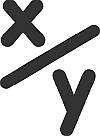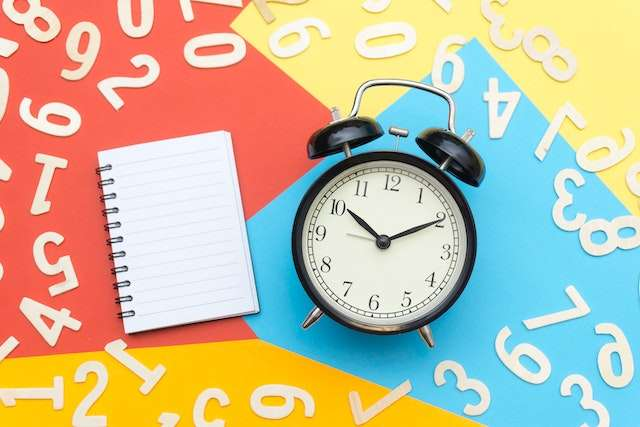# Fraction to Decimal Calculator

Instructions: Use this calculator to convert a given fraction that you provide into a decimal fraction, showing all the steps. Please type in one decimal number in the form below:Type a valid fraction (Ex: 3/2, etc.)

We all know what a fraction is, but sometimes we forget that there is a tight connection between a fraction and a decimal. Indeed, a fraction $$\displaystyle \frac{a}{b}$$ is literally the number $$a$$ divided by $$b$$, so you would expect a decimal as a result.

For example, if you compute take the fraction $$\displaystyle \frac{6}{5}$$ and you interpret it as "6 divided by 5", then when you actually do the calculation you get that 6 divided by 5 is 1.2, which is a decimal number with an integer part.

There is a caveat though: When computing a fraction as a decimal by dividing the numerator by the denominator, not always we will get a decimal as simple as "1.2" like in the previous example. For example, if I compute 1/3 as 1 divided by 3, what I get is 0.33333...., with an endless sequence of 3's.

### How do you convert a fraction to a decimal?

The procedure is simple: for the fraction $$\displaystyle \frac{a}{b}$$ you need to divide $$a$$ by $$b$$. Now, that seems simple, but in reality, we normally use the calculator to do it.

If we were to do the calculation by hand, how to convert fraction to decimal without calculator? There is the neat Euclid remainder theorem, that shows that for two numbers $$a$$ and $$b$$, you have a number $$q$$ (the quotient) and $$r$$ (the remainder) so that $$a = b q + r$$, with $$r < b$$.

For example, if we have $$a = 34$$ and $$b = 12$$, we get $$34 = 2 \cdot 12 + 10$$, so the quotient is 2, and the remainder is 10. Using this algorithm recurrently on the obtained remainder, we continue until the remainder is zero.

### Fractions and Recurring decimals

The process described above does not necessarily end with a zero remainder at some point, because we can find a recurrent decimal, like for example would be case with $$\displaystyle \frac{1}{3}$$

How do we know when we will end up with a recurring decimal?. Well, interestingly, it depends on the denominator: If the prime factors of the decomposition of the denominator are only 2 or 5, or if the only prime is 2, or if the only prime is 5, then the decimal found from a fraction will have and ending digit (will be non-recurrent).

For example, in the case of $$\displaystyle \frac{1}{3}$$, the denominator is 3, and 3 is a prime number, so the denominator has a prime that is not 2 nor 5 in its decomposition, so then we will get a recurring (repeating) decimal

## This Fraction to decimal converter

This calculator will provide the corresponding decimal associated to the fraction provided, and it will assess whether or not the decimal is recurring or not, by analyzing the prime decomposition of the denominator.• Using a decimal could be more concrete, because it is a number
• The problem decimals though is that it can be cumbersome to express recurrent decimals
• Indeed, for the recurring decimal 0.3333... with infinite 3's, we may need to device a way of making it really clear that the sequence of 3's does not end
• With fractions, on the other hand, it is trivial to express recurrent decimals, such as "1/3" for 0.33333......

### More about fractions and percentages

We typically have to work and convert back an forth between decimal and percentages, and fractions as well. Going from fraction to decimal is common, but you would sometimes be interested in going from fraction to percent, and as we know, there is a tight equivalence between percent and decimals.

Also, with this decimal to fraction calculator you can conduct the reverse process of starting with a decimal and arriving to a fraction.

Also, the process of converting from decimal to percentage, and percent to decimal is a very common one. For example, when dealing with rates, and we see that the rate is $$r = 0.04$$, we immediately see that as $$r = 4\%$$, which is simply obtained by multiplying the decimal by 100.

Depending on the use you have for it, you may want to use this fraction to percent calculator, as sometimes you will prefer to see the association of the fraction directly to the percentage.### Example: Conversion of Fraction to Decimal

Question: Calculate the fraction $$\displaystyle\frac{33}{75}$$ as a decimal.

### Fraction to decimal question 2

Question Express 3/81 as a decimal. Is it recurrent?

### Fraction to decimal question 3

Question Convert $$\displaystyle\frac{4597784}{2323453498}$$ as a decimal. Is it recurrent?